Technical Article

# The Skin Effect in Cylindrical and Rectangular Conductors: Eddy Currents and Current Crowding

August 27, 2023 by Dr. Steve Arar

## The distribution of AC currents in real conductors with finite dimensions and circular or rectangular cross-sections is non-uniform. This article will discuss Faraday's law and how AC currents create eddy currents and current crowding in real conductors.

A time-varying current has a nonuniform distribution across the cross-sectional area of the conductor. To approximate the high-frequency resistance of a conductor, we can assume that the whole current flows uniformly in a layer of one skin depth just below the conductor surface. This approximation is actually achieved for a special case where the conductor is a half-space.

In practice, real conductors have finite dimensions and might have a circular or rectangular cross-section. The question to be asked is whether or not the results obtained for a conductive half-space can be applied to other types of wire.

### Current Distribution in Cylindrical Conductors

We can solve Maxwell’s equations for a good conductor to find the following differential equation for the current density J:

$$\nabla ^2 J = j \omega \mu \sigma J$$

##### Equation 1.

If you’re rusty on vector calculus concepts, the intimidating symbol ∇2 (Del squared) is called the Laplacian operator. Put simply, the Laplacian operator is a generalization of the second derivative concept in spaces with more than one dimension. It is given by:

$$\nabla ^2 = \frac{\partial^2 }{\partial x^2} + \frac{\partial^2 }{\partial y^2} + \frac{\partial^2 }{\partial z^2}$$

##### Equation 2.

Equation 1 describes the current distribution in a good conductor. It is valid for both a conductive half-space and a wire with a circular cross-section. However, the solutions we obtain for these two types of media are completely different. For a conductive half-space, the current density is a simple exponentially decaying sinusoidal function (if we assume that we’re dealing with a plane wave). But what about a cylindrical conductor?

From your experience in other fields of physics that involve a cylindrical base, you might have correctly guessed that the answer to Equation 1 should include Bessel functions when the wire has a circular cross-section. This is not good news for us engineers who are always trying to develop a simple model for different phenomena. Bessel functions are useful in modeling a broad range of physics problems, from heat conduction in a cylindrical object to describing the vibrations of a thin circular membrane such as a drumhead. However, they can be difficult to visualize and obviously are much less straightforward than a simple exponentially decaying sine wave.

Due to the complexities of these functions, we won’t go through the mathematical details of the analysis and only look at the results presented in the book “Fields and Waves in Communication Electronics” by Simon Ramo. Figure 1 shows the normalized magnitude of the current distribution across the cross-section of a 1-mm-diameter round wire at four different frequencies.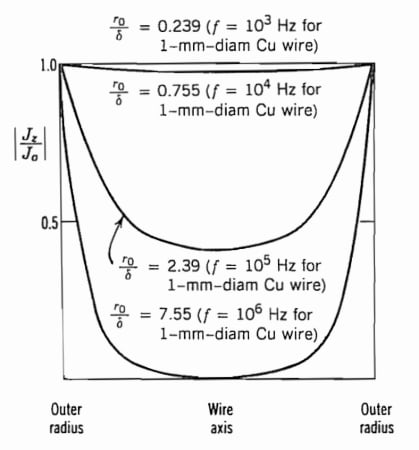##### Figure 1. Normalized magnitude of the current distribution in a circular wire. Image used courtesy of S. Ramo

The parameter r0 in the above graph denotes the wire’s radius. At a frequency (f) of 1 kHz, the skin depth is about 4.2 times larger than the radius of the conductor (or equivalently r0/δ = 0.239). As you can see, the current distribution is almost uniform in this case.

As the frequency increases, the skin depth decreases, and the ratio r0/δ increases from 0.239 at 1 kHz to 7.55 at 1 MHz. Note that even for r0/δ=2.39, the current density at the center of the wire is almost half that at the surface of the conductor. This is not consistent with the simplified description of the skin effect stating that the current density reduces to e-1=0.37 of its surface value at a depth of δ.

Figure 2 compares the actual current distributions for r0/δ=2.39 and r0/δ=7.55 with the exponentially decaying distribution of the current density (which corresponds to wave propagation in a conductive half-space). As you can see, the results of the half-space case can be used to approximate the actual current distribution in a round wire only if the conductor’s radius of curvature is much larger than the skin depth.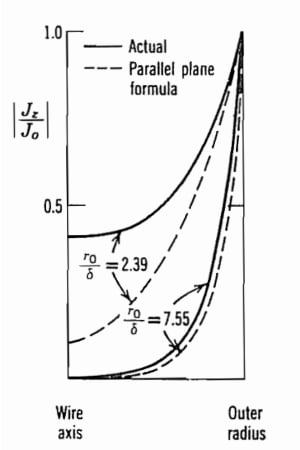##### Figure 2. Actual current distribution versus parallel plane formula. Image used courtesy of S. Ramo

As a rule of thumb, if all radii of curvature and thicknesses of the conductor are at least 3–4 times greater than the skin depth, we assume that a given conductor resembles a semi-infinite block . Thus far in this two-part series, we have relied on solving Maxwell’s equations to describe some of the most important features of the skin effect. A deeper (and maybe more useful) insight into this effect can be developed by noting how Faraday’s law of induction can produce eddy currents inside the conductor. Armed with this insight, we can have a better understanding of how different interconnects behave.

### Faraday’s Law of Induction and Eddy Currents

According to Faraday’s law, a changing magnetic field induces an electromotive force (and hence a current) in a conductive wire. The simple and familiar experiment in Figure 3(a) illustrates the basic idea of this law. By bringing a magnet closer to a loop of wire, an emf is induced in the wire, which consequently produces an induced current.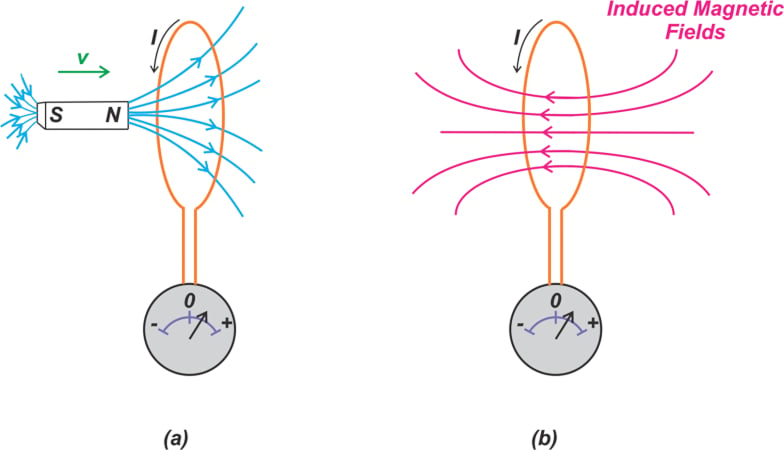##### Figure 3. Changing magnetic fields induce currents in conductors

The induced emf is directly proportional to the time rate of change of the magnetic flux through the circuit. Besides, the induced current tends to oppose the change in the original magnetic flux that produced the induced current in the first place (Lenz’s law).

Figure 3(b) shows the induced magnetic flux when the magnet is brought closer to the loop of wire. The induced magnetic field is directed such that it tries to keep the original magnetic flux from changing.

#### What Are Eddy Currents?

Just as a changing magnetic field can induce a current in a loop, it can also produce circulating currents in a bulk piece of metal when it is placed in a changing magnetic field. These circulating currents are known as eddy currents, as illustrated in Figure 4.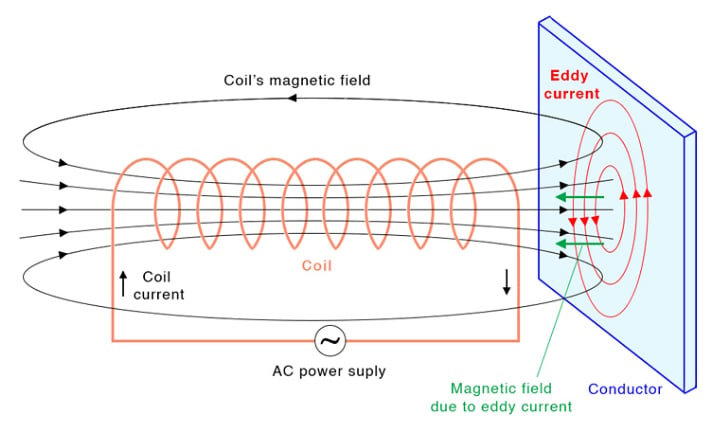##### Figure 4. Creation of eddy currents in a conductive plane. Image used courtesy of Sciencefacts

Again note the direction of the induced current. Assuming that the coil’s magnetic field increases in the depicted direction, a current in a counterclockwise direction is induced to produce a magnetic field that opposes changes in the original magnetic field from the coil.

### Eddy Currents Within a Current-Carrying Conductor

When current flows through a wire, it produces a magnetic field both inside the wire and outside of it. With an AC current, we have a time-varying magnetic field inside the wire, which according to Faraday’s law, will produce eddy currents inside the wire. This is illustrated in Figure 5 below.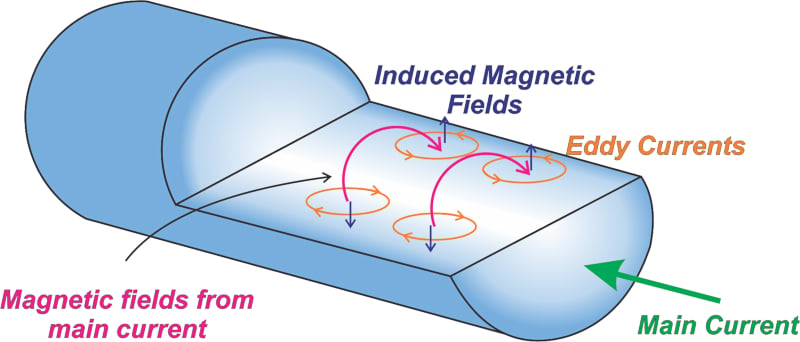##### Figure 5. AC currents induce eddy currents with a wire.

As can be seen, the eddy currents flow in the direction of the main current near the surface of the conductor, but they flow in the opposite direction in the inner parts of the conductor. Thus, the overall current flowing near the surface of the conductor increases, whereas the current flowing through deeper layers of the conductor decreases.

### Current Crowding in Conductors

It should be noted that certain surfaces of a conductor might be more effective in carrying AC current than others. To understand this, recall from our discussion above that changing magnetic fields cause the skin effect. Now, if the magnetic fields are stronger at a particular surface of the conductor, we can expect that surface to carry a larger portion of the current. For example, consider the cross-section of a microstrip line shown in Figure 6.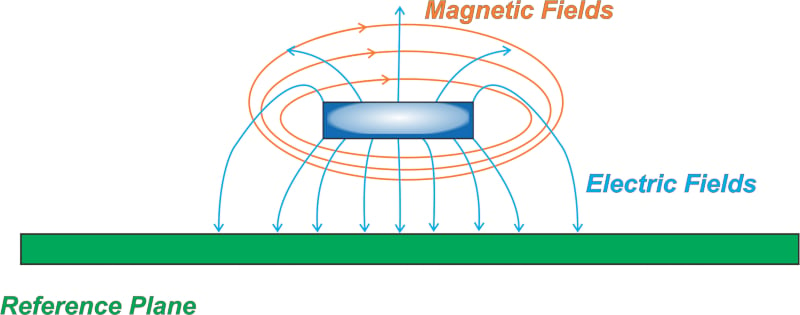##### Figure 6. Cross-section of a microstrip line conductor over a reference plane.

Figure 6 shows the general pattern of the electric and magnetic fields as well as the rough current distribution in a microstrip line. Please note that this is not a simulated result. It only shows the rough behavior!

Did you notice that the magnetic fields are more concentrated between the bottom surface of the trace and the ground plane? As a result, the microstrip’s bottom surface carries more current than its top surface. For example, about 60% of the current might be concentrated in the darkly shaded region of the line’s cross-sectional area. The phenomenon of current crowding at a particular surface due to the effect of an adjacent current-carrying conductor is known as the proximity effect.

#### Current Crowding in Parallel Wires

A similar effect is observed when dealing with two parallel cylindrical wires (Figure 7). In this figure, the direction of current flow in the two conductors is opposite, and hence the magnetic field between the wires is the strongest. As a result, the current crowds on the surfaces facing each other.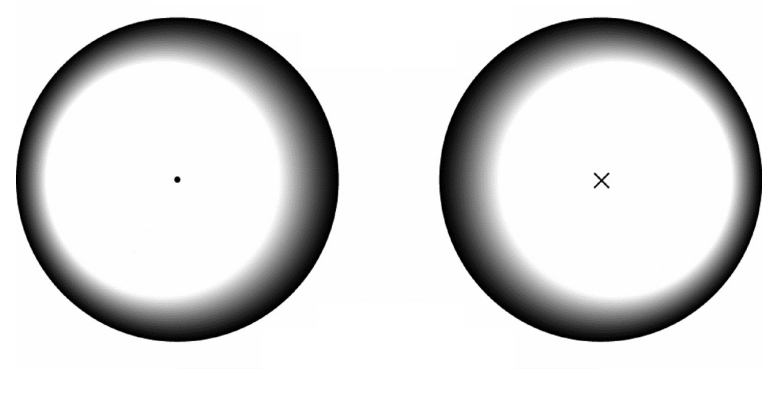#### Current Crowding in Rectangular Conductors

It’s interesting to note that even for an isolated wire (with no proximity effect), all surfaces of a conductor might not carry the same amount of current. For example, the current distribution of a thin, wide, rectangular conductor is shown in Figure 8. As you can see, the current density at the vertical surfaces is greater than the horizontal surfaces. This is due to having stronger magnetic fields at these surfaces.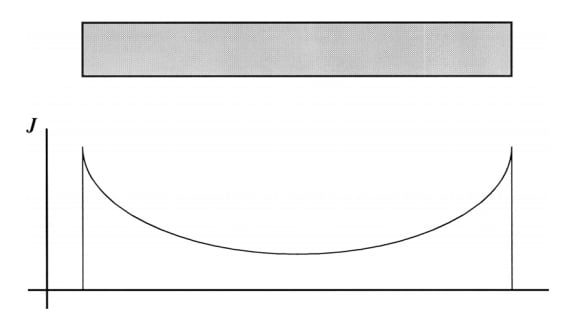#### Current Crowding in Square Conductors

From the results shown above for a thin rectangular conductor, you might be able to guess what the current disribution looks like for a square conductor Figure 9 shows current crowding in a conductor with a square cross-section. The current density is highest in the four corners.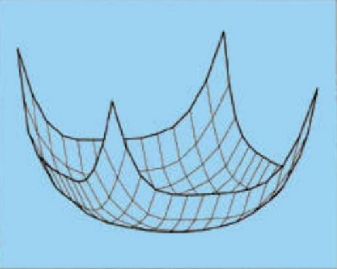### Don't Forget the Effects of Frequency on Current Crowding

As we discussed near the beginning of this article, the current distribution and the skin depth are a function of the frequency. Naturally then, the current crowding also depends on the frequency of the AC signal. There will be no current crowding for DC signals, and it will increase with increasing frequency (up to a certain point).

With current crowding, conductors suffer from secondary effects that need to be considered in real applications. The effective resistance of a conductor can be higher than if we assume a constant current distribution. The current crowding can also cause increased heating and stress in the conductors. As you create new designs that use time-varying signals, it is good practice to always consider the potential impact of eddy currents and current crowding on circuit performance.

Unless otherwise noted, all images used courtesy of the author.•sjgallagher2 August 29, 2023
•W4GHV September 01, 2023